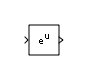# Math Function

•HDL Coder / Math Operations

## 描述

Math Function 模块执行许多常见的数学函数。

`exp`

`eu`

`exp`
`log`

`ln u`

`log`
`2^u`

`2u`

`2.^u`
（请参见`power`

`10^u`

`10u`

`10.^u`
（请参见 `power`

`log10`

`log u`

`log10`
`magnitude^2`

`|u|2`

`real(u).^2 + imag(u).^2`
（请参见 `real``imag``power`

`square`

2 次幂

`u2`

`u.^2`
（请参见 `power`

`pow`

`sign(u)*|u|v`（默认值，仅适用于偶数阶根）或 `uv`

`power`
`conj`

`ū`

`conj`

`1/u`

`1./u`
（请参见 `rdivide`

`hypot`

`(u2+v2)0.5`

`hypot`
`rem`

`rem`
`mod`

`mod`
`transpose`

`uT`

`u.'`
（请参见数组与矩阵运算

`hermitian`

`uH`

`u'`
（请参见数组与矩阵运算

`exp`

`log`

`2^u`

`10^u`

`log10`

`magnitude^2`

`square`

`pow`

`conj`

`hypot`

`rem`

`mod`

`transpose`

`hermitian`

### 牛顿-拉夫逊倒数算法方法

`$f\left(x\right)=\frac{1}{x}-a.$`

Simulink® 选择 $0<{x}_{0}<\frac{2}{a}$ 范围内的一个初始估计值，因为这是函数的收敛域。

`${x}_{i+1}={x}_{i}-\frac{f\left({x}_{i}\right)}{f\text{'}\left({x}_{i}\right)}={x}_{i}+\left({x}_{i}-a{x}_{i}{}^{2}\right)={x}_{i}.\left(2-a{x}_{i}\right)$`

$f\text{'}\left(x\right)$ 是函数 $f\left(x\right)$ 的导数。

### 数据类型支持

`exp`

`log`

`2^u`

`10^u`

`log10`

`magnitude^2`

`square`

`pow`

`conj`

`hypot`

`rem`

`mod`

`transpose`

`hermitian`

## 端口

### 输出

#### 使用牛顿-拉夫逊方法的 `reciprocal`

`fi` (value, 0, word_length, fraction_length)

`fi` (value, 0, word_length, word_length–fraction_length–1)

`fi` (value, 1, word_length, fraction_length)

`fi` (value, 1, word_length, word_length–fraction_length–2)

## 参数

### 常设

#### 编程用法

 模块参数：`Operator` 类型：字符向量 值：`'exp' | 'log' | '2^u' | '10^u' | 'log10' | 'magnitude^2' | 'square' | 'pow' | 'conj' | 'reciprocal' | 'hypot' | 'rem' | 'mod' | 'transpose' | 'hermitian'` 默认值：`'exp'`

`reciprocal` 函数的算法方法，指定为完全牛顿-拉夫逊。要使用牛顿-拉夫逊逼近方法计算倒数，请选择牛顿-拉夫逊。否则，请选择完全

#### 编程用法

 模块参数：`AlgorithmType` 类型：字符向量 值：`'Exact' | 'Newton-Raphson'` 默认值：`'Exact'`

• on - 通过将输入信号绝对值乘以输入信号的符号来计算幂。

• off - 计算实际输入值的幂。如果第一个输入为负值，第二个输入为偶数阶根，则返回 `nan`

#### 编程用法

 模块参数：`SignedPower` 类型：字符向量 值：`'on' | 'off' | ` 默认值：`'on'`

`exp`, `log`, `2^u`, `10^u`, `log10`, `square`, `pow`, `reciprocal`, `conjugate`, `transpose`, `hermitian`

`real`

`complex`

`real`

`complex`

`real`

`error`

`complex`

`complex`

`magnitude squared`

`real `

`complex`

`real`

`real`

`real`

`real`

`complex`

`complex`

`hypot`, `rem`, `mod`

`real`

`complex`

`real`

`error`

`real`

`error`

`complex`

`error`

#### 编程用法

 模块参数：`OutputSignalType` 类型：字符向量 值：`'auto' | 'real' | 'complex'` 默认值：`'auto'`

#### 依存关系

• 函数设置为倒数

• 算法方法设置为牛顿-拉夫逊

#### 编程用法

 模块参数：`Iterations` 类型：字符向量 值：`'3' | scalar` 默认值：`'3'`

#### 编程用法

 模块参数：`SampleTime` 类型：字符串标量或字符向量 默认值：`"-1"`

### 信号属性

#### 编程用法

 模块参数：`OutMin` 类型：字符向量 值：`'[ ]'`| 标量 默认值：`'[ ]'`

#### 编程用法

 模块参数：`OutMax` 类型：字符向量 值：`'[ ]'`| 标量 默认值：`'[ ]'`

• 继承数据类型的规则，例如继承: 通过反向传播继承

• 内置数据类型的名称，例如 `single`

• 数据类型对象的名称，例如 `Simulink.NumericType` 对象

• 计算结果为数据类型的表达式，例如 `fixdt(1,16,0)`

#### 依存关系

• 要启用此参数，请将函数设置为 magnitude^2方波倒数

• 对于 magnitude^2square，当输入是小于单精度的浮点数据类型时，Inherit: 从内部规则继承输出数据类型取决于 Inherit floating-point output type smaller than single precision 配置参数的设置。当编码数据类型所需的位数小于编码单精度数据类型所需的 32 位时，数据类型小于单精度。例如，`half``int16` 小于单精度。

#### 编程用法

 模块参数：`OutDataTypeStr` 类型：字符向量 值：`'Inherit: Inherit via internal rule` | `'Inherit: Same as first input'` | `'Inherit: Inherit via back propagation'` | `'double'` | `'single'` | `'half'` | `'int8'` | `'uint8'` | `'int16'` | `'uint16'` | `'int32'` | `'uint32'` | `'int64'` | `'uint64'` | `'fixdt(1,16)'` | `'fixdt(1,16,0)'` | `'fixdt(1,16,2^0,0)'` | `''` 默认值：`'Inherit: Same as first input'`

#### 编程用法

 模块参数：`LockScale` 类型：字符向量 值：`'off' | 'on'` 默认值：`'off'`

#### 编程用法

 模块参数：`RndMeth` 类型：字符向量 值：`'Ceiling' | 'Convergent' | 'Floor' | 'Nearest' | 'Round' | 'Simplest' | 'Zero'` 默认值：`'Floor'`

`int8`（有符号 8 位整数）数据类型可以表示的最大值为 127。任何大于此最大值的模块运算结果都会导致 8 位整数溢出。如果选中此复选框，模块输出将在达到 127 时饱和。类似地，模块输出将在达到最小输出值 -128 时饱和。

`int8`（有符号 8 位整数）数据类型可以表示的最大值为 127。任何大于此最大值的模块运算结果都会导致 8 位整数溢出。清除该复选框时，软件会将导致溢出的值解释为 `int8`，这可能产生意外结果。例如，以 `int8` 表示的模块结果 130（二进制 1000 0010）为 -126。

#### 编程用法

 模块参数：`SaturateOnIntegerOverflow` 类型：字符向量 值：`'off'` | `'on'` 默认值：`'on'`

## 模块特性

 数据类型 `Boolean` | `double` | `fixed point` | `half` | `integer` | `single` 直接馈通 `是` 多维信号 `是` 可变大小信号 `是` 过零检测 `否`